STRESS AND STRAIN PRODUCED IN A BAR BY ITS OWN WEIGHT

# 1STRESS AND STRAIN PRODUCED IN A BAR BY ITS OWN WEIGHT

## 1.1Problem definition

Verify the total elongation and cross section area of a vertical prismatical bar at axial force and of dead weight.

## 1.2 References (Timoshenko)

S. Timoshenko, Strength of Material, Part I, Elementary Theory and Problems, 3rd Edition, D. Van Nostrand Co., Inc., New York, NY, 1956, Chapter 1.5, pg. 15.

Steel beam with given axial stress. The following listed values are used:

• Load at lower end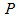• Length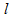• Working Stress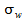• Density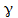• Modulus of Elasticity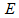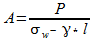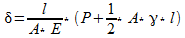Where:

 Variable Description Unit Used Value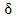Total elongation inch 2,5271Length of the prismatic beam feet/ inch 720/ 8640Working Stress lbs/ inchÂ² 10000Modulus of Elasticity lbs/ inchÂ² 30000000Load lbf 70000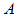Cross section inchÂ² 9,2722Density lbs/ ftÂ³ 490

Table 1: Overview of the used variables

## 1.3 Model description (ROHR2)

The ROHR2 model consists of a 720 feet long carbon steel beam. The only parameter necessary for this test case is the cross section which is given by Timoshenko as9,27 inchÂ² â‰™ 59,82 cmÂ². In the model an equivalent pipe dimension of 88 mm x 38,3 mm (diameter x wall thickness) is taken. The loads applied in thisexample is a point load at the lower end (2) of 70000 lbf and dead load. In the load case (Lc) dead load the gravitational acceleration is taken into account.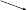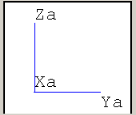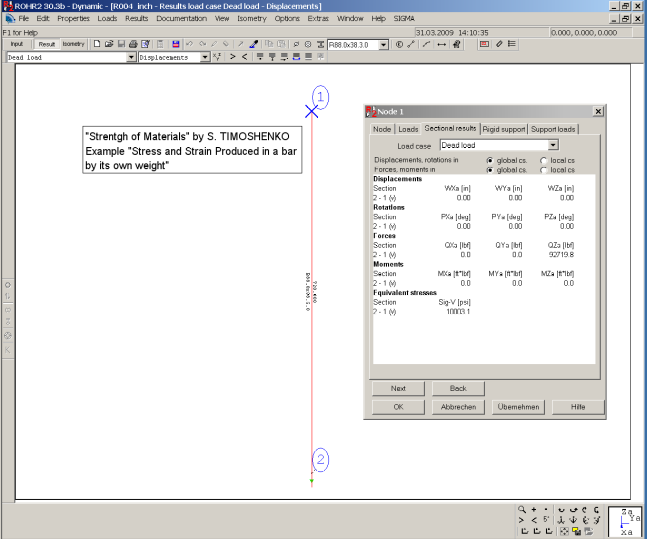Figure 1: Rohr2 model with sectional results (equivalent stress)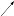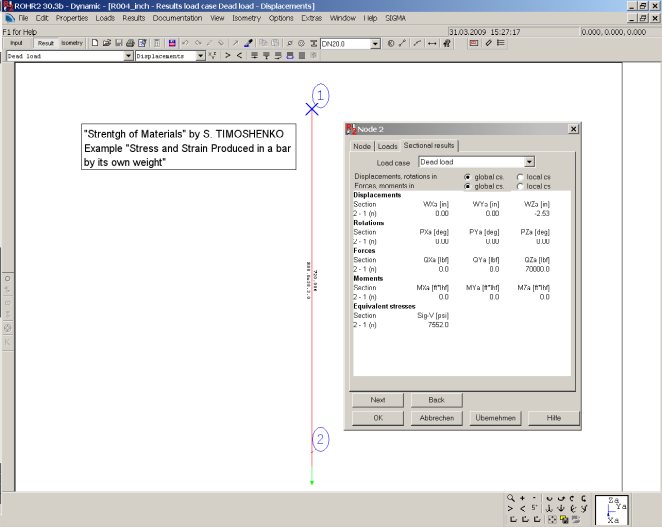Figure 2: Rohr2 model with sectional results (displacements)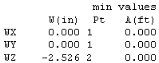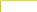Figure 3: Î´ from the Lc Dead Weight

## 1.4 Result comparisons

 Value Length [inch] Reference (Timoshenko) [inch] Rohr2 [inch] Difference [%]8640 2,5271 2,5260 <0,05

Table 2: Comparison of the elongation at the lower end

 Value Cross section (Timoshenko) [inchÂ²] Cross section (Rohr 2) [inchÂ²] Difference [%]9,2722 9,2691 <0,04

Table 3: Comparison between demand and used cross section

## 1.5 Conclusion

The results are very close to the reference by Timoshenko. The small difference is due to the number of digit entered for the cross section. The results precision is excellent.

## 1.6 Files

R004_inch.r2w

R004_mm.r2w

R2_stresses_4.ods

SIGMA Ingenieurgesellschaft mbH www.rohr2.com